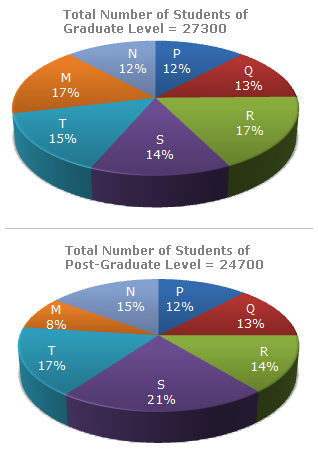# Data Interpretation - Pie Charts - Discussion

The following pie-charts show the distribution of students of graduate and post-graduate levels in seven different institutes in a town.2.

What is the ratio between the number of students studying at post-graduate and graduate levels respectively from institute S?

 [A]. 14 : 19 [B]. 19 : 21 [C]. 17 : 21 [D]. 19 : 14

Explanation:

 Required ratio = (21% of 24700) = (21 x 24700) = 19 . (14% of 27300) 14 x 27300 14

 Tejash Bhavsar said: (Aug 20, 2013) I would like to understand that what is actual method to calculate ratio, Here I would like to add that 21*24700/14*27300 = to come different figure. So please let me know that should be ideally calculated?

 Wemimo Prosper said: (Oct 29, 2013) How or what quick method can we use or know that it is 273 that will divide 5187 and 3822 to give us 19:14. Quickest way of solving ratio?

 Gopal Thakur said: (Jul 20, 2014) Divide 5187 and 3822 by 3 you will get 1729 and 1274. Then add units of each number i.e.,1+7+2+9 = 19 and 1+2+7+4 = 14. Thus Ratio between PG and Grad of institute S is 19:14.

 Sushil said: (Oct 17, 2015) Please explain way to calculate the ratios in more detail.

 Anuj said: (Sep 3, 2016) 24700 * 21/100 : 27300 * 14/100 = 19 : 14.

 Sarika said: (Jul 20, 2017) Divide 5187 and 3822 by 3 you will get 1729 and 1274. Then add units of each number i.e.,1+7+2+9 = 19 and 1+2+7+4 = 14. Thus Ratio between PG and Grad of Institute S is 19:14. @Gopal. From where does this 3 comes from?

 Manish Sonwane said: (Sep 8, 2017) From where you take this 5187 and 3822 values? Explain clearly.

 Gauri said: (Oct 18, 2017) @ GOPAL, SARIKA & MANISHA. P.G from Institute S = 24700*(21/100) = 247*21 = 5187. Grad. from institute S = 27300*(14/100) = 273*14 = 3822. P.G./Graduate = 5187/3822 {DIVIDE 5187 & 3822 BY 3} = 1729/1274 {DIVIDE 1729 &1274 BY 91} = 19/14.

 Sushmitha said: (Apr 27, 2018) How 3 came? @Gauri.

 Renuka Arvind Chitale said: (Jun 8, 2018) How the 21 comes we write 21% of 24700 so we do 21/100*24700 instead of this we doing 21*24700 how this possible? Please explain.

 Prakash said: (Jul 6, 2018) @Renuka. Here, 100 and 100 get cancelled.

 Vidhi said: (Dec 29, 2018) From where 3 is coming? Please explain the step.

 Vijaya said: (Dec 8, 2019) 3 is divisible in both D numbers 5187 &3822.

 Gema said: (Jun 29, 2021) @Gauri. How 3 came? Explain.